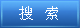说明：双击或选中下面任意单词，将显示该词的音标、读音、翻译等；选中中文或多个词，将显示翻译。 您的位置：首页 -> 句库 -> 超线性收敛 1. Local Q-superlinear Convergence of a Modified BFGS Algorithm一类修正BFGS算法的局部超线性收敛性 2. The authors also prove that the method has superlinear convergence rate.证明了此法具有全局收敛性和局部超线性收敛性. 3. A Class of Generalized Quasi-Newton Algorithms with Superlinear Convergence;具有超线性收敛性质的一类广义拟牛顿算法 4. Research on the Superlinearly Convergent Algorithms for Optimization with Equilibrium Constraints 均衡约束优化具有超线性收敛性算法的研究 5. A Superlinearly Convergent SQP Algorithm for Infinte Discretized Problems From Semi-infinite Programming 离散半无限规划的一个超线性收敛的SQP算法 6. A Superlinearly Convergent SSLE Algorithm for Optimization Problem with Linear Complementarity Constraints; 线性互补约束优化问题一个超线性收敛的序列线性方程组算法 7. A Superlinearly Convergent Norm-Relaxed Method of Strongly Sub-Feasible Directions for Nonlinearly Constrained Optimization; 非线性约束最优化超线性收敛的模松弛强次可行方向法 8. The Superlinear Convergence of Quasi-Newton Methods Based on the New Quasi-Newton Equation; 基于新拟牛顿方程的拟牛顿法的超线性收敛性分析 9. A Superlinearly Convergent Norm-Relaxed Method of Feasible Directions for Finely Discretized Problems from Semi-Infinite Programming; 半无限规划离散化问题超线性收敛的模松弛可行方向法 10. A Superlinearly Convergent Strongly Sub-Feasible SQP Algorithm for Constrained Optimization Without Strict Complementarity; 约束优化无严格互补的超线性收敛SQP强次可行算法 11. A SUPERLINEARLY CONVERGENT FSSLE ALGORITHM FOR INEQUALITY CONSTRAINED OPTIMIZATION WITH QUASI-REGULARITY 不等式约束优化拟正则条件下的超线性收敛FSSLE算法 12. The Global Superconvergence of Finite Element Method for a Kind of Nonlinear Hypobolic Equation 非线性拟双曲方程有限元方法的整体超收敛 13. The Convergence Theorems of Iteration Methods for Nonlinear Equations and Convergence Rate Estimate; 非线性方程迭代法的收敛定理及收敛率估计 14. Some Ultra-convergent Derivative Recovery Schemes for Piecewise Linear Interpolation;分片线性插值的几种强超收敛的导数后处理格式 15. Asymptotic Expansion and Super Convergence of a Kind of Quasilinear Parabolic and Hyperbolic Equations Using Generalized Finite Element Method; 一类拟线性抛物与双曲方程广义有限元方法的渐近展式和超收敛 16. Asymptotic Expansion and Superconvergence of Generalized Finite Element Method for Quasilinear Equations; 拟线性方程广义有限元方法的渐进展式和超收敛 17. The Global Superconvergence of Finite Element Method for a Kind of Strongly Nonlinear Parabolic Integro-differential Equations 一类完全非线性抛物积分微分方程有限元方法的整体超收敛 18. Superconvergence Analysis of Nonconforming FEM Approximation of A Quasilinear Elliptic Problem of Nonmonotone Type 非协调元逼近非单调型拟线性椭圆问题的超收敛分析 ©2011 dictall.com